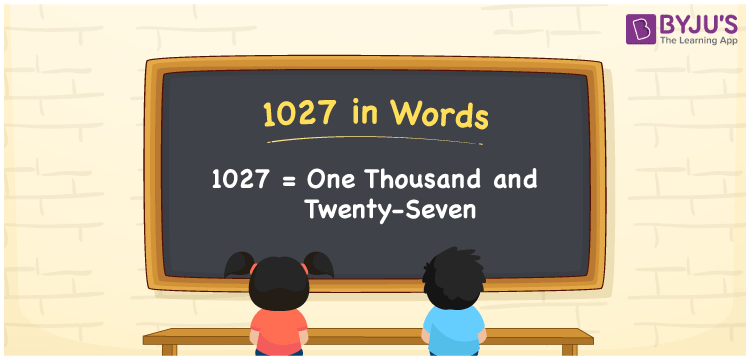# 1027 in Words

We can write 1027 in words as One Thousand and Twenty-Seven. For example, if the cost of a book is Rs. 1027, then you can express, “The cost of the book is Rupees One Thousand and Twenty-Seven”. 1027 is a cardinal number as it denotes a certain amount. We can convert the number 1027 into words effortlessly using a place value system. This article provides a complete explanation about the conversion of the number 1027 into words.

 1027 in Words One Thousand and Twenty-Seven One Thousand and Twenty-Seven in numerical form 1027

## 1027 in English Words

Generally, we represent the numbers in words using the letters of the English alphabet. Therefore, the number 1027 in words is written as One Thousand and Twenty-Seven.## How to Write 1027 in Words?

1027 is a four-digit number. Hence, we require a table of 4 columns to depict its place value chart. The place value chart for 1027 and its expanded form is given below.

 Thousands Hundreds Tens Ones 1 0 2 7

Hence, we can write the expanded form as:

1 x Thousand + 0 x Hundred + 2 x Ten + 7 x One

= 1 x 1000 + 0 x 100 + 2 x 10 + 7 x 1

= 1000 + 0 + 20 + 7

= 1000 + 20 + 7

= 1027

= One Thousand and Twenty-Seven

Therefore, 1027 in words is written as One Thousand and Twenty-Seven

Interesting way of writing 1027 in words

1 = One

10 = Ten

102 = One Hundred and Two

1027 = One Thousand and Twenty-Seven

Thus, the word form of the number 1027 is One Thousand and Twenty-Seven

1027 is a natural number that is one less than 1028 and one greater than 1026

• 1027 in words – One Thousand and Twenty-Seven
• Is 1027 an odd number? – Yes
• Is 1027 an even number? – No
• Is 1027 a perfect square number? – No
• Is 1027 a perfect cube number? – No
• Is 1027 a prime number? – No
• Is 1027 a composite number? – Yes

## Frequently Asked Questions on 1027 in Words

Q1

### How do you write 1027 in words?

1027 in words is written as One Thousand and Twenty-Seven.
Q2

### Simplify 1000 + 27, and express in words.

Simplifying 1000 + 27, we get 1027. Therefore, the number 1027 in words is One Thousand and Twenty-Seven.
Q3

### Convert One Thousand and Twenty-Seven in numbers.

One Thousand and Twenty-Seven in numbers is 1027.